# Problem 53810. List the Beatriz numbers

Write a function to find integers n less than or equal to an input value m that solve the equation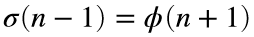. The function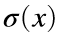gives the sum of divisors of x; for example,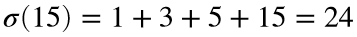. The totient function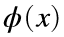counts numbers less than x that are relatively prime to x. For example,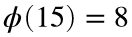because 1, 2, 4, 7, 8, 11, 13, and 14 are relatively prime to 15; that is, the greatest common divisor of 15 and each of the eight numbers is 1.
An oblique hint is that I call solutions to“Beatriz numbers” in honor of a good friend and former co-worker of EmilyR and JessicaR. The natural search will not help, unless you are interested in combinatorics related to Higham’s conjecture.

### Solution Stats

66.67% Correct | 33.33% Incorrect
Last Solution submitted on Oct 08, 2022

### Community Treasure Hunt

Find the treasures in MATLAB Central and discover how the community can help you!

Start Hunting!